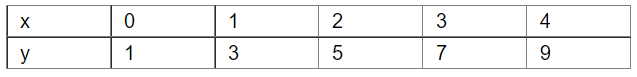# Plot a line graph for the variables x and y , where y = 2x + 1.

Question:

Plot a line graph for the variables x and y , where y = 2x + 1.

Using the graph, find the value of (i) y, when x = 5 and (ii) x, when y = 13.

Solution:

We have the following table for y = 2x+1